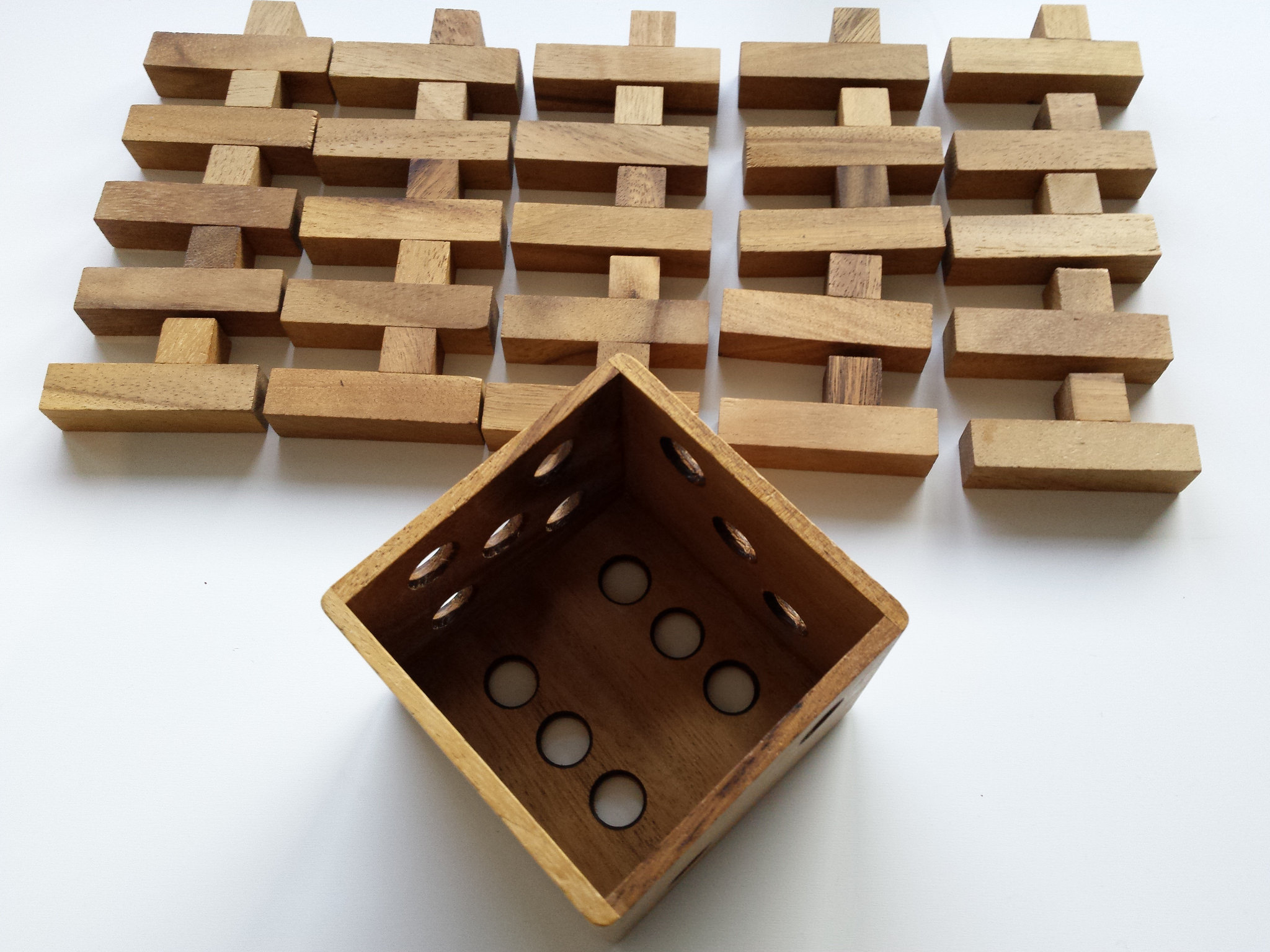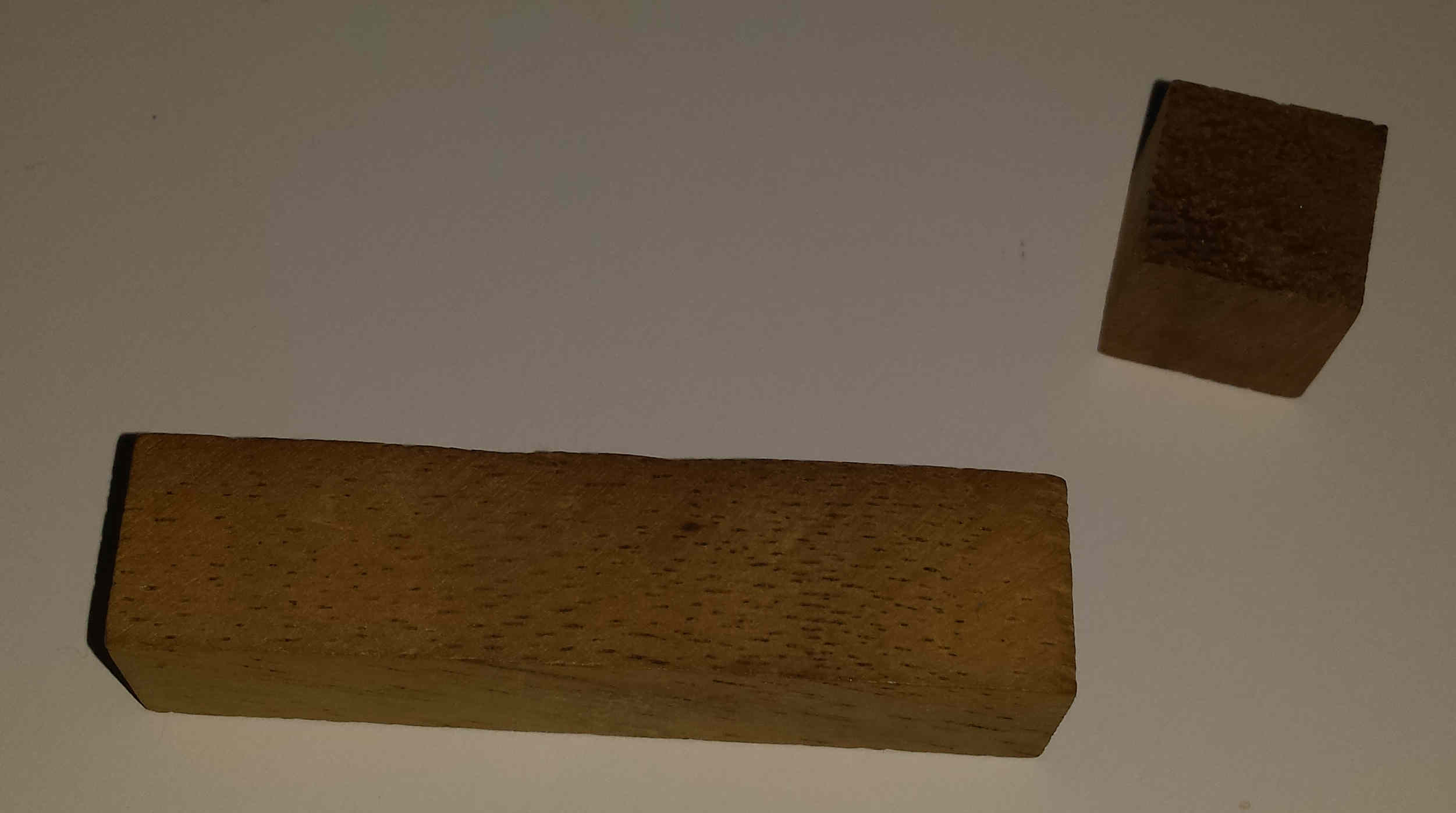# Packing 25 Y pentacubes in a 5x5x5 box

There is some confusion in the internet concerning the following puzzle:For example, here a nice sketch of a solution is given while the number of solutions mentioned is largely understimated. Also previous versions of this article in Wikipedia overstimated the number of first possible moves.

The first pentacube can be put in 960 different ways inside the empty box (the y pentacube has 24 orientations which can be repeated 2x4x5 times). There are 60672 different ways to pack the box including all rotation and mirror packings. A cube is invariant under 48 transformations that combine reflections and rotations. Therefore the 5x5x5 box can be packed in 1264=60672/48 fundamental ways. This is also thanks to the fact that there is no single packing featuring any of the 48 possible symmetries.

This was computed using the famous algorithm X from Knuth. I used the python version from Ali Assaf. After finding unique cover I removed the symmetric solutions so that only fundamental packing remain. My codes are in python: ypentacubes.py, readsolutions.py. The first code ypentacubes.py finds the 60672 packing. It takes about 2 days to run. The second code readsolutions.py takes the output and removes all symmetric solutions, leaving the fundamental 1264 solutions: unique.dat.

# The puzzle with one broken y pentacube

Some time ago one ypentacube broke into an I tetracube and a monocube:It is expected that thanks to this the puzzle is now easier to assemble. My codes are a bit slow for this but one friend of mine used BurrTools to find all possible solutions. He obtained that there are 11196736 total solutions and 699807 removing rotations and mirror symmetries. This makes the broken puzzle about 500 times easier to solve than the original one, nevertheless most humans are likely unable to solve either puzzle without computer assistance. Note that many of the solutions must feature some symmetry since 11196736/48=233265.33 < 699807. More intriguingly 699807/233265.33 = 3.000047 or 11196736./ 699807 = 15.9997, why?